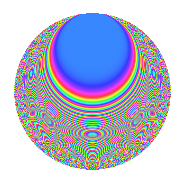# Properties

 Label 315.2.uLevel 315 Weight 2 Character orbit u Rep. character $$\chi_{315}(59,\cdot)$$ Character field $$\Q(\zeta_{6})$$ Dimension 88 Newform subspaces 1 Sturm bound 96 Trace bound 0

# Related objects

## Defining parameters

 Level: $$N$$ = $$315 = 3^{2} \cdot 5 \cdot 7$$ Weight: $$k$$ = $$2$$ Character orbit: $$[\chi]$$ = 315.u (of order $$6$$ and degree $$2$$) Character conductor: $$\operatorname{cond}(\chi)$$ = $$315$$ Character field: $$\Q(\zeta_{6})$$ Newform subspaces: $$1$$ Sturm bound: $$96$$ Trace bound: $$0$$

## Dimensions

The following table gives the dimensions of various subspaces of $$M_{2}(315, [\chi])$$.

Total New Old
Modular forms 104 104 0
Cusp forms 88 88 0
Eisenstein series 16 16 0

## Trace form

 $$88q - 38q^{4} - 6q^{5} + 12q^{6} - 6q^{9} + O(q^{10})$$ $$88q - 38q^{4} - 6q^{5} + 12q^{6} - 6q^{9} - 6q^{10} - 12q^{14} - 6q^{15} - 26q^{16} - 12q^{19} + 6q^{20} - 12q^{21} - 42q^{24} - 2q^{25} + 12q^{26} + 6q^{29} - 18q^{30} - 6q^{31} + 12q^{34} - 36q^{36} - 6q^{41} + 84q^{44} - 12q^{45} - 18q^{46} + 10q^{49} + 30q^{50} - 6q^{51} - 48q^{54} - 90q^{56} - 6q^{59} + 54q^{60} + 12q^{61} - 8q^{64} + 54q^{65} + 78q^{66} - 60q^{69} - 30q^{70} + 12q^{75} + 48q^{76} + 8q^{79} + 69q^{80} + 42q^{81} + 120q^{84} - 7q^{85} - 72q^{89} - 33q^{90} + 20q^{91} - 6q^{94} - 93q^{95} - 12q^{96} + 30q^{99} + O(q^{100})$$

## Decomposition of $$S_{2}^{\mathrm{new}}(315, [\chi])$$ into newform subspaces

Label Dim. $$A$$ Field CM Traces $q$-expansion
$$a_2$$ $$a_3$$ $$a_5$$ $$a_7$$
315.2.u.a $$88$$ $$2.515$$ None $$0$$ $$0$$ $$-6$$ $$0$$

## Hecke Characteristic Polynomials

There are no characteristic polynomials of Hecke operators in the database